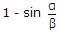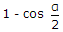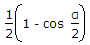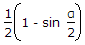# Civil Engineering - Waste Water Engineering

### Exercise :: Waste Water Engineering - Section 4

46.

Pick up the incorrect statement from the following :

 A. The process of decomposing the organic matter under controlled anaerobic conditions, is called sludge digestion B. Sludge digestion is carried out in sludge tank C. The gases produced in sludge digestion process, contain 75% carbon dioxide D. The gases produced in sludge digestion process, contain 75% methane.

Explanation:

No answer description available for this question. Let us discuss.

47.

Pick up the correct statement from the following :

 A. The circular section of sewers provides maximum hydraulic mean depth B. The circular sewers are provided for separate sewerage system C. The circular sewers work efficiently if the sections run at least half full D. Two sewers of different shapes are said to be hydraulically equivalent if they discharge at the same rate while running full on the same grade E. All the above.

Explanation:

No answer description available for this question. Let us discuss.

48.

You are asked to design sewer pipes of diameters 0.4 m to 0.9 m at maximum flow, you will assume the sewer flow running at

 A. full depth B. half full C. two third full D. three fourth full.

Explanation:

No answer description available for this question. Let us discuss.

49.

The ratio of depths at partial flow with central angle α and at full flow of a sewer, is

 A.B.C.D.E.Explanation:

No answer description available for this question. Let us discuss.

50.

For sewer mains of 0.5 to 1 m diameter, the ratio of maximum daily sewage flow to the average daily sewage flow is assumed

 A. 1.5 B. 2 C. 2.5 D. 3